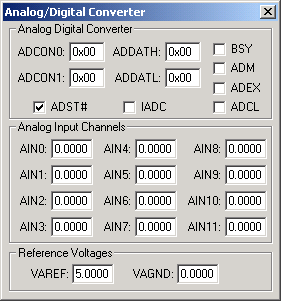Products Download Events Support Videos All Product Families ARM7, ARM9, and Cortex-M3 Products C16x, XC16x, and ST10 Products C251 and 80C251 Products Cx51 and 8051 Products Modified Anytime In the Last 7 Days In the Last 14 Days In the Last 30 Days In the Last 90 Days In the Last 6 Months

# Peripheral Simulation

For Infineon SAB 80C517A — A/D Converter (10-bit, 12 channels)

Simulation support for this peripheral or feature is comprised of:

• Dialog boxes which display and allow you to change peripheral configuration.
• VTREGs (Virtual Target Registers) which support I/O with the peripheral.

These simulation capabilities are described below.

### Analog/Digital Converter DialogThe Analog/Digital Converter dialog displays and configures the A/D converter. The controls in this dialog are separated into several logical groups.

Analog/Digital Converter Group

• ADDATH (A/D Converted Data - High byte) contains the most significant 8-bits of the 10-bit A/D conversion result.
• ADDATL (A/D Converted Data - Low byte) contains the least significant 2-bits of the 10-bit A/D conversion result.
• BSY (Busy Flag) is hardware controlled and is set when an A/D conversion is in progress.
• ADM (A/D Mode) is set to trigger a new A/D conversion automatically (Continuous Mode). If reset, only 1 value is converted (Single Conversion Mode). Resetting this bit stops A/D conversion when the current conversion completes.
• ADEX External Start) when set, uses the falling edge of P4.0 (ADST) to start an A/D conversion.
• ADCL (A/D Conversion Clock Ratio) used with ADCL0 to determine the prescaler ratio for the A/D conversion clock. This bit is reset by default
• IADC (A/D Converter Interrupt Request Flag) is set by the hardware when an A/D conversion completes. It must be reset by software.
• ADST# (External Start Pin) reflects the value of P4.0 (ADST) signal level.

• AIN0-AIN3 (Analog Input Channels 0-3) are used for setting voltage input to the ADC. These 4 inputs are alternate functions for Port pins P7.0-P7.3. The text box displays the analog voltage value for each input channel.
• AIN4-AIN7 (Analog Input Channels 4-7) are used for setting voltage input to the ADC. These 4 inputs are alternate functions for Port pins P7.4-P7.7. The text box displays the analog voltage value for each input channel.
• AIN8-AIN11 (Analog Input Channels 8-11) are used for setting voltage input to the ADC. These 4 inputs are alternate functions for Port pins P8.0-P8.3. The text box displays the analog voltage value for each input channel.

Reference Voltages Group

• VAREF (A/D Reference Voltage) contains the maximum voltage value allowed for A/D conversion. When the analog input value exceeds this value, the digital result is 0x3FF.
• VAGND (A/D Reference Ground) contains the minimum voltage value allowed for A/D conversion. When input voltage is below this value, the digital result is 0x0000.

### AINx VTREGData Type: float

```AIN0 = 0.000  /* Analog Input 0 = 0.000 volts */
```

You may also enter the analog input voltages using the A/D Converter Dialog which is accessable from the Peripherals menu. You may create a debugger signal function to periodically change the value of the A/D input. The following signal function increases the A/D Channel 1 input voltage by 0.1 volts each second.

```signal void AIN1_sig (void) {
float f;

for (f = 0.0; f < 5.0; f += 0.1)
{
swatch (1.0);                  // Delay 1 second
AIN1 = f;                      // Set AIN1 Voltage
}
}
```

### VAGND VTREGData Type: float

The VAGND VTREG contains the A/D Reference Ground (the minimum voltage allowed for A/D conversions). When an A/D input voltage is below this value, the digital result is 0x0000.

### VAREF VTREGData Type: float

The VAREF VTREG contains the A/D Reference Voltage (the maximum voltage allowed for A/D conversions). When the analog input value exceeds this value, the digital result is 0x3FF.